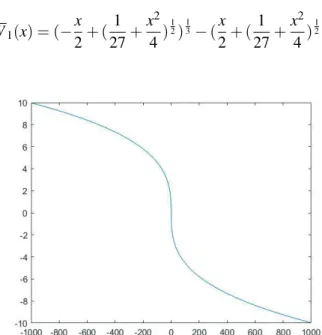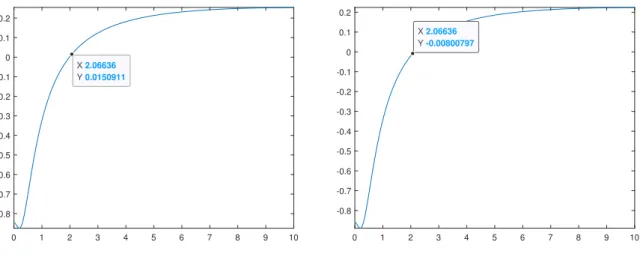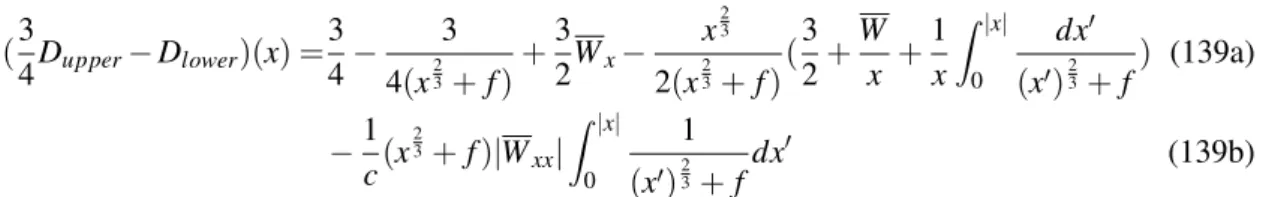• 검색 결과가 없습니다.

# Singularity formation of Euler equations

N/A
N/A
Protected

Share "Singularity formation of Euler equations"

Copied!
68
0
0
더 보기 ( 페이지)

전체 글

The main idea of ​​the proof is to consider the general 2D Euler solution as a small perturbation of the 1D Burgers equation. According to Collot-Ghoul-Masmoudi , one of the self-similar solutions is linearly stable, and all other Burgers solutions will be drawn from that solution at the singularity point. Using the idea of ​​Buckmaster-Shkoller-Vicol , we can show that the 1D Euler solution is also in C13.

## Definition of a singularity and a shock

The right side of this equation is inC1, therefore we get W∈C2. By repeating the same procedure, we arrive at the conclusion W ∈C∞. The converse of the claim follows through the inverse function theorem. Since the Burgers equation has spatial translation invariance, so do steady self-similar Burgers. Since the Burgers equation has time-translation invariance, so do steady self-similar Burgers.Figure 1: The graph of W 1 (x) Hence, the taylor series of ∂ x W 1 (x) is

## Riemann invariants

The main point we argue is the global well-posedness of the smooth solution and its uniqueness.

No vacuum for 1D Euler

## Singularity Theorem

Set r=∂xw1, and define the new characteristics x(θ,t) satisfying dx(θdt,t)=λ1,and x(θ,0) =θ. Then the equation becomes Therefore, the C1 norm of ρ,u is bounded in [0,T]×R. Therefore, from an a priori assessment, the solution is well positioned globally. This implies that ∂xw1 blows, so the rate C1 of ρ and u explode at the same time T.

In this proof, ∂xw blows up at the singularity time when the second condition is satisfied. Therefore, we can guess that the derivative of some values ​​corresponding to the Riemann invariants in 2D Euler will also diverge. Also, in Appendix B, we will see the regularity of the 1D Euler solution at the singularity time using the idea of ​​Buckmaster-Shkoller-Vicol .

In this chapter we will see the singularity formation of 2D isentropic compressible Euler equations, introduced by Buckmaster-Shkoller-Vicol . For the shock formation of multidimensional Euler equations, the various works on non-rotating flow (zero vorticity) exist with the idea that the equation turns into second-order quasilinear wave equation. Therefore, in Buckmaster-Shkoller-Vicol  we focus on the flow with non-zero vorticity, and not on a perturbation of plane waves.

We will consider the 1D Euler solution as a small perturbation of a Burgers solution, which will be considered a purely azimuthal 2D Euler solution.

## Polar form of 2D Euler Equation

To find a relation to 1D equation, let's set a=0 for a moment so that the solution of 2D is independent of r. We can see that under this condition the equations (72b), (72c) are perfectly equivalent to 1D Euler system. To see the variables that are likely to blow up, let's turn all the variables into Riemann invariants using 1D idea.

Through our iteration in (82), we can see that all the variables {ck}and {λk} are indeed bounded. Since {λk} are the escape velocities, we now have a domain G such that all points starting in G cannot escape G. One such G is a hexagonal region bounded by the two vertical lines t=±α and the four steeply sloping lines x±β =±Kt. Our idea is by choosing small enough α, which reduces the range of ​​Ukm,Xkm.

Like 2nK. Because the bounds become tighter as α goes to 0, the infinite series represent a convergent solution to the Cauchy problem. We can repeat the same process by proving local existence if the corresponding K is uniformly defined. But we have excluded the||w||L∞ and||z||L∞ terms from the necessary condition because these remain finite if a∈Lt1L∞x, and also the||∂θa||L∞ term.

If we set up a new vortex using our new variables, ω= ωP = 2b−∂Pθa, it satisfies∂tω+b∂θω =αaω. It is a transport equation and the vortex cannot break up, so the force term must be finite.

## Self-similar variables

These new relations seem viable at first, but correspond to Burgers' self-similar solution, U(t,θ) = (−t)2i1Wi(x)withi=1, sincee−s2 = (τ(t)− t) 12. Although the domain iθ is a compact domain T, the domain of W,Z,A can be extended to R. We will prove it later. As we mentioned before, we need the initial condition (104) to share the same initial condition as Wi. Proposition 7 Changing the variables of the 1D Burgers equation using similar variables and substituting τ˙=0,ξ˙ =c,κ=c, we get (∂s−12)U+ (32x+U)Ux =0 with W as solution.

Therefore, in 2D Euler equation, τ˙=0,ξ˙ =c,κ=c is the condition equivalent to the transformation of the equation into 1D Burgers equation.

## Our goal

It means that ∂θwf first inT∗. To ensure that T∗ is the smallest singularity time, we need the conditions∂θa,∂θz<∞ast→T∗. But before we show it, let's check that our solutions satisfy the conditions of positive density and nonzero vorticity all the time. a). Also, since P(θ,t)≥(α2inft|w−z|)α1, to guarantee P(θ,t)>0, we need a uniform lower bound c of P satisfying|w−z | ≥c>0. Here, we will define the following initial data conditions for ease of future calculations. From here on, we will denote W =W1, where W1 was a solution of Burgers' consistent self-similar equation.

We deliberately make the equation in the form of the last term to use the second maximum principle in Appendix A. x0)23+f, which is the kernel notation in the second maximum principle. Since the second term is non-negative at x∗(s) and the first term is equal to dsν(φ(x,s),s) for the characteristicφ satisfying ∂ φ∂s =U(x,s), we obtain. For this we need to check: the final initial data, the final velocity characteristics and the final term of the force.

Now we will check whether all terms corresponding to force and speed terms are finite or not. To do this, let's see if both conditions can be satisfied at the same time. Since we want to see W as a solution of Burgers equation at γ=3, we hope to extend the domain to R.

Let's rearrange the inequalities we needed for our purpose and prove it using the bootstrap method.

Assumptions on the initial datum

Bootstrap assumptions

## Closing the assumptions

However, since finer bounds are needed for W than the previous assumptions, we will focus on a toy problem to get an idea of ​​the calculation. Now, to evaluate the damping term of (207c), split the middle terms into two, so that one contains only the functions we already know, such as W, and the other has the remainder, because the middle terms can be new depreciation term. 208a) We do not know the exact value of the second term, but we want a situation where the first term has a positive lower bound large enough to cover the second term, so that it can have a positive lower bound of the total amortization term. For this, we will define a new variable V(x,s) =g(x,s)W˜x with some functionsg(x,s). Here, g will be determined later in the process of establishing a positive lower bound. can use the relations ˜Wx=Vg,W˜xx=Vx−. The first term becomes a damping term of V, and the second obviously does not.

We will find a limit of V, but because F =0, the limit will be constant by the maximum principle. At this point, we see that we cannot use the first maximum principle because the lower bound goes to 0 as x decreases to 0. Since|F|=0 and K=0, their conditions satisfy the second maximum principle. 1+x2)12. Therefore, to have a constant bound on ˜V, additional initial guesses on the partial derivatives of W are required.

To satisfy the next condition ||V˜(x,s)||L∞(|x|

Therefore, any open set that satisfies the rest second conditions and the third one, which is transformed to satisfy the first condition, will satisfy all the initial conditions of W.

Bootstrap method

## Maximum principles

Also, there exists a minimum time s∗ and its corresponding x∗ such that |f(x∗,s∗)|= 3m4 because f is a continuous function (if not, the transport equation is not well defined.).

## Perturbed form of 1D Burgers

When z=0 in (76), we proved that w∈C13 is at the singularity time when the initial date W is in a special small neighborhood of W in C4. So we are interested in the solution (w,z) (252) that satisfies w6=0,z6=0. They are not solutions of (252), but we can show the decay rate of Win in the same way as 2D Euler. Like 2D Euler, we can change the variables using self-similar variables and modulations. According to Theorem 2, when the derivatives of one Riemann invariant are negative for some point, the 1D Euler solution explodes at the same singularity time T , and before such T the solution is well defined.

To show the regularity of w at the singularity time, we changed the equation of w to a new variableν, and applied the second maximum principle to find upper bound ofν. Finally, by the relation between W and w, we obtained w∈C13. In that idea, we have never used (76b) and (76c), so we do not need to modify the proof at all, for the proof of 1D Euler solution. This substitution does not disturb the limit of power term, therefore we can apply the second maximum principle as in chapter 4.3.

The proof of 2D uses Proposition 7, to say that w and z would not affect the divergence of e∂θz. For this, we will make the adequate property corresponding to Proposition 7.

## Closing bootstrap assumption

This was because the proof of 2D Euler solution almost ignores the modulations and derivatives of Z and A due to their sufficiently small limits. In this paper, we have studied that the initial data for solutions of 1D Burgers, 1D Euler, 2D Euler whose profile at the time of singularity is inC13 are in an open set iC4. For this, we first introduced the 1D Burgers self-similar solution W, and saw that 1D Euler, 2D Euler equations can be expressed as a perturbed form of 1D Burgers, in Appendix B and Chapter 4.

And then we explained that the general solution of these equations has the same decay rate as W, so we proved that the difference between Wx and the spatial derivative of the general solution is bounded. First, we changed the 2D isentropic compressible Euler to polar form and tried to find a solution independent of r to use the property solution of the 1D Euler equation. The new a,b,P equation coincided with the 1D Euler equation at a=0 and we found that the a=0 condition is equivalent to γ=3.

At this point, since one of the Riemannian invariants of the 1D Euler solution has a diverging derivative at the singularity point, we could guess that the same would happen on the 2D Euler generala6=0 conditions. In particular, the fact that the regularity of w at the singularity point becomes C13 like it is a new achievement in . Wang, “The Cauchy problem for the Euler equations for compressible fluids,” in Handbook of Mathematical Fluid Dynamics.

Finally, I would like to thank my family, who always encouraged me to continue my studies, and Hyunsoo Kang, who always supported me for seven years.

수치참조

관련 문서

The risk averse investors who want to find out ‘safe haven’ that are designed to protect their wealth from extreme market volatility would not be happy to the

The extent of destruction of microorganisms in PEF processing depends mainly on the electric field strength of the pulses and treatment time.. These are the two most

ASDAL( Advanced Ship Design Automation Laboratory) (http://asdal.snu.ac.kr) Ship design, Ship Types, 2008.3.. LNG선의 화물창 시스템(Cargo Containment System) –

캐나다 New Brunswick의 SMR 건설에 관심을 보이고 있으며, CNSC의 pre-licensing vendor design review에 참여하고 있다.. 당시는 발전용 원자로 개발이 최초로

In 2015, New South Wales Aboriginal Affairs, Department of Education (‘NSW Aboriginal Affairs’) engaged the Australian Institute of Health and Welfare (AIHW) to provide an overview

Thanks to lower prices for local rice, wage-to-rice terms of trade improved in Lofa Country (Liberia), Nzerekore (Guinea) and in Eastern Province (Sierra Leone).

* Section 8 of the Banking Reform Act requires the Treasury to establish an independent review of the operation of the ring-fencing legislation by 2021 (within 2 years of the rest

The Australian Institute of Health and Welfare (AIHW) annually collects data from organisations funded by the Australian Government to provide one or more of the following

In addition to offering technical assistance to FAO projects on capacity development for pest and pesticide management, Israel’s agency for International Development regularly

언어 선택하기

웹사이트는 선택한 언어로 번역됩니다.

기타 언어